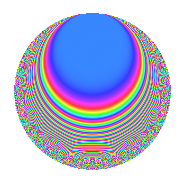# Properties

 Label 201.3.h.aLevel 201 Weight 3 Character orbit 201.h Analytic conductor 5.477 Analytic rank 0 Dimension 22 CM No

# Related objects

## Newspace parameters

 Level: $$N$$ = $$201 = 3 \cdot 67$$ Weight: $$k$$ = $$3$$ Character orbit: $$[\chi]$$ = 201.h (of order $$6$$ and degree $$2$$)

## Newform invariants

 Self dual: No Analytic conductor: $$5.47685331364$$ Analytic rank: $$0$$ Dimension: $$22$$ Relative dimension: $$11$$ over $$\Q(\zeta_{6})$$ Sato-Tate group: $\mathrm{SU}(2)[C_{6}]$

## $q$-expansion

The dimension is sufficiently large that we do not compute an algebraic $$q$$-expansion, but we have computed the trace expansion.

 $$\operatorname{Tr}(f)(q) =$$ $$22q + 26q^{4} - 15q^{7} - 66q^{9} + O(q^{10})$$ $$\operatorname{Tr}(f)(q) =$$ $$22q + 26q^{4} - 15q^{7} - 66q^{9} + 6q^{10} + 30q^{11} - 78q^{12} - 27q^{13} + 6q^{14} - 6q^{15} - 58q^{16} - 8q^{17} + q^{19} - 12q^{20} - 15q^{21} + 14q^{22} - q^{23} - 56q^{25} + 71q^{26} - 75q^{28} + 42q^{29} + 18q^{30} + 120q^{31} - 105q^{32} + 30q^{33} - 24q^{34} + 3q^{35} - 78q^{36} + 13q^{37} + 108q^{38} + 27q^{39} + 82q^{40} - 159q^{41} + 189q^{44} + 372q^{46} - 35q^{47} - 174q^{48} - 40q^{49} + 285q^{50} - 24q^{51} - 212q^{55} - 113q^{56} + 3q^{57} + 136q^{59} - 12q^{60} + 63q^{61} - 150q^{62} + 45q^{63} - 284q^{64} - 20q^{65} + 94q^{67} + 154q^{68} - 3q^{69} - 41q^{71} - 16q^{73} + 441q^{74} - 390q^{76} - 84q^{77} - 213q^{78} - 615q^{79} + 267q^{80} + 198q^{81} - 302q^{82} - 154q^{83} + 75q^{84} + 633q^{85} + 221q^{86} - 126q^{87} + 410q^{88} + 56q^{89} - 18q^{90} + 272q^{91} + 636q^{92} + 120q^{93} + 588q^{95} - 105q^{96} - 441q^{97} + 780q^{98} - 90q^{99} + O(q^{100})$$

## Embeddings

For each embedding $$\iota_m$$ of the coefficient field, the values $$\iota_m(a_n)$$ are shown below.

For more information on an embedded modular form you can click on its label.

Label $$a_{2}$$ $$a_{3}$$ $$a_{4}$$ $$a_{5}$$ $$a_{6}$$ $$a_{7}$$ $$a_{8}$$ $$a_{9}$$ $$a_{10}$$
97.1 −3.30238 + 1.90663i 1.73205i 5.27046 9.12871i 0.881143i 3.30238 + 5.71988i 1.91999 + 1.10851i 24.9422i −3.00000 −1.68001 2.90987i
97.2 −2.55387 + 1.47448i 1.73205i 2.34817 4.06715i 9.46283i 2.55387 + 4.42343i −6.24108 3.60329i 2.05347i −3.00000 13.9527 + 24.1668i
97.3 −2.11074 + 1.21863i 1.73205i 0.970137 1.68033i 1.83558i 2.11074 + 3.65590i 4.69421 + 2.71020i 5.02011i −3.00000 −2.23690 3.87442i
97.4 −2.09110 + 1.20729i 1.73205i 0.915119 1.58503i 7.66078i 2.09110 + 3.62188i −6.04816 3.49191i 5.23908i −3.00000 −9.24881 16.0194i
97.5 −0.443170 + 0.255864i 1.73205i −1.86907 + 3.23732i 5.57502i 0.443170 + 0.767593i 3.11708 + 1.79965i 3.95982i −3.00000 1.42645 + 2.47068i
97.6 0.0720773 0.0416138i 1.73205i −1.99654 + 3.45810i 1.32016i −0.0720773 0.124841i 1.88003 + 1.08543i 0.665245i −3.00000 −0.0549368 0.0951533i
97.7 0.658194 0.380008i 1.73205i −1.71119 + 2.96386i 4.20783i −0.658194 1.14002i −7.58591 4.37973i 5.64113i −3.00000 1.59901 + 2.76957i
97.8 1.57301 0.908176i 1.73205i −0.350432 + 0.606966i 5.97048i −1.57301 2.72453i 6.73988 + 3.89127i 8.53843i −3.00000 5.42225 + 9.39162i
97.9 2.08753 1.20524i 1.73205i 0.905201 1.56785i 5.75683i −2.08753 3.61572i −7.36527 4.25234i 5.27798i −3.00000 −6.93836 12.0176i
97.10 2.82230 1.62946i 1.73205i 3.31026 5.73353i 4.04184i −2.82230 4.88837i 7.82069 + 4.51528i 8.54003i −3.00000 −6.58601 11.4073i
97.11 3.28813 1.89840i 1.73205i 5.20788 9.02031i 3.86882i −3.28813 5.69521i −6.43146 3.71321i 24.3594i −3.00000 7.34458 + 12.7212i
172.1 −3.30238 1.90663i 1.73205i 5.27046 + 9.12871i 0.881143i 3.30238 5.71988i 1.91999 1.10851i 24.9422i −3.00000 −1.68001 + 2.90987i
172.2 −2.55387 1.47448i 1.73205i 2.34817 + 4.06715i 9.46283i 2.55387 4.42343i −6.24108 + 3.60329i 2.05347i −3.00000 13.9527 24.1668i
172.3 −2.11074 1.21863i 1.73205i 0.970137 + 1.68033i 1.83558i 2.11074 3.65590i 4.69421 2.71020i 5.02011i −3.00000 −2.23690 + 3.87442i
172.4 −2.09110 1.20729i 1.73205i 0.915119 + 1.58503i 7.66078i 2.09110 3.62188i −6.04816 + 3.49191i 5.23908i −3.00000 −9.24881 + 16.0194i
172.5 −0.443170 0.255864i 1.73205i −1.86907 3.23732i 5.57502i 0.443170 0.767593i 3.11708 1.79965i 3.95982i −3.00000 1.42645 2.47068i
172.6 0.0720773 + 0.0416138i 1.73205i −1.99654 3.45810i 1.32016i −0.0720773 + 0.124841i 1.88003 1.08543i 0.665245i −3.00000 −0.0549368 + 0.0951533i
172.7 0.658194 + 0.380008i 1.73205i −1.71119 2.96386i 4.20783i −0.658194 + 1.14002i −7.58591 + 4.37973i 5.64113i −3.00000 1.59901 2.76957i
172.8 1.57301 + 0.908176i 1.73205i −0.350432 0.606966i 5.97048i −1.57301 + 2.72453i 6.73988 3.89127i 8.53843i −3.00000 5.42225 9.39162i
172.9 2.08753 + 1.20524i 1.73205i 0.905201 + 1.56785i 5.75683i −2.08753 + 3.61572i −7.36527 + 4.25234i 5.27798i −3.00000 −6.93836 + 12.0176i
See all 22 embeddings
 $$n$$: e.g. 2-40 or 990-1000 Embeddings: e.g. 1-3 or 172.11 Significant digits: Format: Complex embeddings Normalized embeddings Satake parameters Satake angles

## Inner twists

This newform does not have CM; other inner twists have not been computed.

## Hecke kernels

This newform can be constructed as the kernel of the linear operator $$T_{2}^{22} - \cdots$$ acting on $$S_{3}^{\mathrm{new}}(201, [\chi])$$.